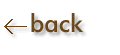Powers of Ten:  Multiplication and Division

MULTIPLICATION

Multiply the numbers.

Add the exponents for the power of ten.

Examples:   (3.0201 x 102) x (2.0 x 101)  =  6.0 x 103

(Use correct number of significant figures.  Use correct scientific notation)

Note:  If a power of ten is not expressed, it is understood to be 100, which equals 1.  Thus, 4.0 is understood to be 4.0 x 100.

DIVISION

Divide the numbers as in any division problem.

Subtract the denominator power of ten exponent from the numerator power of ten exponent.

Examples:   (6.0830 x 104) / (1.73 x 102)  =  3.52 x 102

(Use correct number of significant figures.  Use correct scientific notation)